Next: Update Rules Up: Cost Function Previous: Terms of the Cost

## Posterior Mean and Variance of f(s(t))

This section describes how to compute the posterior mean and variance of the outputs fk(s(t)) of the MLP network. Ordinarily the inputs, weights and biases of an MLP network have fixed values. Here the inputs s(t), weights A, B and the biases a, b have posterior distributions which means that we also have a posterior distribution of the outputs. One way to evaluate the posterior mean and variance is to propagate distributions instead of fixed values through the network. Whole distributions would be quite tricky to deal with, and therefore we are going to characterise the distributions by their mean and variance only.

The sources have mixture-of-Gaussians distributions for which it is easy to compute the mean and variance: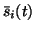=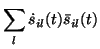(19)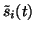=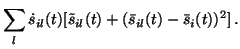(20)

Then the sources are multiplied with the first layer weight matrix A and the bias a is added. Let us denote the result by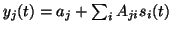. Since the sources, weights and biases are all mutually independent a posteriori, the following equations hold: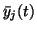=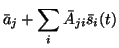(21)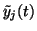=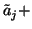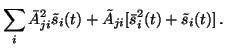(22)

Equation (31) follows from the identity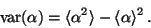(23)

For computing the posterior mean of the output gj(yj(t)) of a hidden neuron, we shall utilise the second order Taylor's series expansion of gj around the posterior meanof its input. This means that we approximate
 gj(yj(t))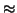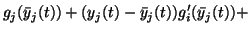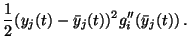(24)

Since the posterior mean of yj(t) is by definition, the second term vanishes when evaluating the posterior mean, while the posterior mean of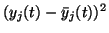is by definition the posterior variance. We thus have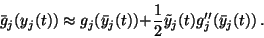(25)

The second order expansion was chosen because those are the terms whose posterior mean can be expressed in terms of posterior mean and variance of the input. Higher order terms would have required higher order cumulants of the input, which would have increased the computational complexity with little extra benefit.

For the posterior variance of gj(yj(t)) the second order expansion would result in terms which need higher than second order knowledge about the inputs. Therefore we shall use the first order Taylor's series expansion which then yields the following approximation for the posterior variance of gj(yj(t)):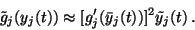(26)

The next step is to compute the mean and variance of the output after the second layer mapping. The outputs are given by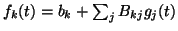. The equation for the posterior mean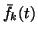is similar to (30):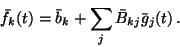(27)

The equation for the posterior variance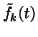is more complicated than (31), however, since si(t) are independent a posterior but gj(t) are not. This is because each si(t) affects several -- potentially all -- gj(t). In other words, each si(t) affects each fk(t) through several paths which interfere. This interference needs to be taken into account when computing the posterior variance of fk(t).

We shall use a first order approximation of the mapping f(s(t)) for measuring the interference. This is consistent with the first order approximation of the nonlinearities gj and yields the following equation for the posterior variance of fk(t):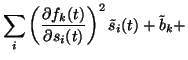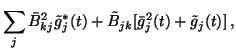(28)

where the posterior means of the partial derivatives are obtained by the chain rule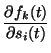=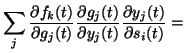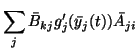(29)

and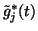denotes the posterior variance of gj(t) without the contribution from the sources. It can be computed as follows: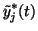=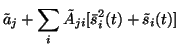(30)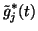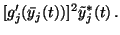(31)

Notice thatappears in (39) and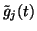appears in (37). These terms do not contribute to interference, however, because they are the parts which are randomised by multiplication with Aji or Bkj and randomising the phase destroys the interference, to use an analogy from physics.Next: Update Rules Up: Cost Function Previous: Terms of the Cost
Harri Lappalainen
2000-03-03